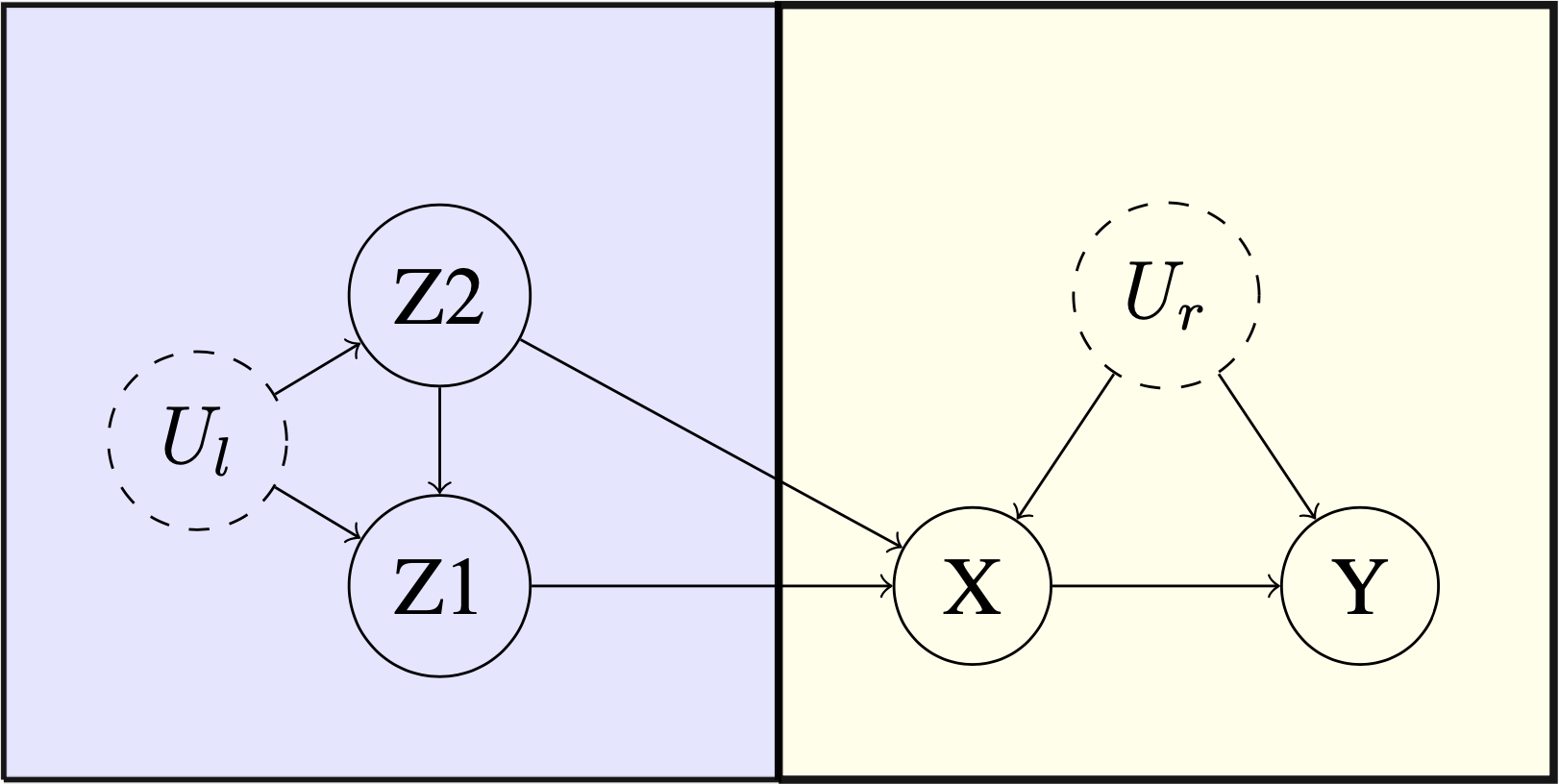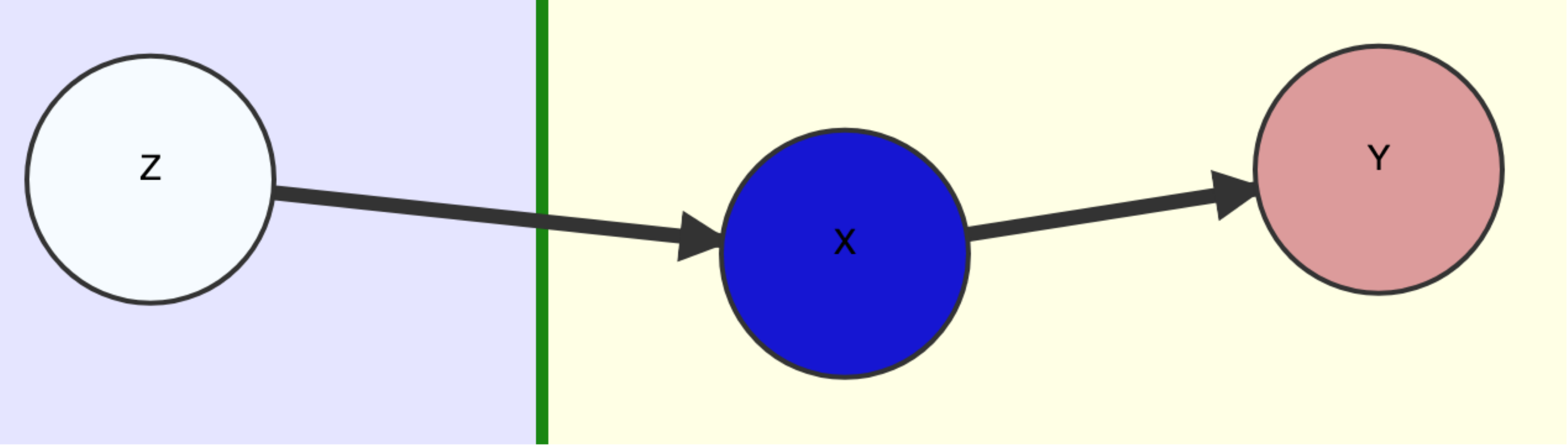# Improving the speed of computing causal bounds

library(causaloptim)

# What is causaloptim?

In summary, causaloptim computes symbolic tight bounds on causal effects. Suppose we have a causal graph $$G$$ in which we want to estimate an effect $$\text{ACE}(X\rightarrow Y)$$. If there is unmeasured confounding of this effect, then it cannot be determined. However, we can still try to find good bounds on it!

Let’s pretend for a while that the confounding was in fact measured, so we have an estimate of its distribution $$q$$. Then, given $$q$$, the effect $$\text{ACE}_{q}(X\rightarrow Y)$$ is identifiable. Now, even though we don’t actually know $$q$$, we surely know something about it; it needs to be consistent with the structure of $$G$$, so it satisfies some constraint, say $$q\in\mathcal{A}$$. With no further assumptions, $$\text{ACE}_{q}(X\rightarrow Y)$$ is valid w.r.t. $$G$$ if and only if we have $$q\in\mathcal{A}$$, so finding tight bounds on $$\text{ACE}(X\rightarrow Y)$$ becomes a constrained optimization problem; simply find the extrema of $$\{\text{ACE}_{q}(X\rightarrow Y):q\in\mathcal{A}\}$$.

For a given estimate of the distribution $$p$$ of our measured variables, we could try a large number of simulations and numerically approximate the bounds, but can we actually solve the problem analytically to get closed form expressions of the bounds in terms of $$p$$? For a certain class of problems, it turns out we can! This is precisely what causaloptim does, and this post will highlight some of its inner workings and recent efficiency improvements. For a tutorial on how to get started using causaloptim, have a look at this post.

# Background

Back in 1995, [Balke & Pearl]1 showed how to do this in a classic example of an experiment with potential non-compliance. More on that shortly, but in summary they showed how to translate their causal problem into a linear programming one and leverage tools from linear optimization to compute the bounds. Balke even wrote a command line program in C++ that computes them, given such a linear program (LP) as input. His program has been used successfully by researchers in causal inference since then, but has had a few issues preventing it from wider adoption among applied researchers.

causaloptim was written to solve these problems. It can handle the generalized class of problems defined in [Sachs & Gabriel & Sjölander]2. It removes the burden of translating the causal problem into an LP and has an intuitive graphical user interface that guides the user through specifying their DAG and causal query. It has until version 0.7.1 used Balke’s code for the optimization. However, for non-trivial instances of the generalized class that causaloptim handles, the original optimization algorithm has not been up to the task.

# Efficiency matters!

Let’s time the following simple enough looking multiple instrument problem.Benchmark Example. Multiple Instruments.

library(causaloptim)
library(igraph)
b <- graph_from_literal(Z1 -+ X, Z2 -+ X, Z2 -+ Z1, Ul -+ Z1, Ul -+ Z2, X -+ Y, Ur -+ X, Ur -+ Y)
V(b)$leftside <- c(1, 0, 1, 1, 0, 0) V(b)$latent <- c(0, 0, 0, 1, 0, 1)
E(b)$rlconnect <- c(0, 0, 0, 0, 0, 0, 0, 0) E(b)$edge.monotone <- c(0, 0, 0, 0, 0, 0, 0, 0)
obj <- analyze_graph(b, constraints = NULL, effectt = "p{Y(X = 1) = 1} - p{Y(X = 0) = 1}")

Using causaloptim version 0.7.1 to bound $$\text{ACE}(X\rightarrow Y)$$ we got (with a 3.3 GHz Quad-Core Intel Core i5; your mileage may vary)

system.time(oldbnds <- optimize_effect(obj))
#>     user  system  elapsed
#> 31093.57   47.02 61368.22

Note the times (in seconds)! 😱 Our CPU spent almost $$9$$ hours working on this! 🤖 And we spent more than $$17$$ hours waiting! 😴 Correctness is not enough; having to wait until the next day is a bad user experience. Using causaloptim version 0.8.0 however, we get

system.time(newbnds <- optimize_effect_2(obj))
#>  user  system  elapsed
#> 0.139   0.001    0.140

Now this is acceptable! 😊 Of course, we need to verify that the results are the same. It is difficult to see upon visual inspection that they are the same, because the vertices are returned in a different order (and there are lots of them). Instead we can randomly generate some probability distributions that satisfy the graph, and compute the bounds and compare the results. First, we create the R functions that compute the bounds:

eval_newbnds <- interpret_bounds(newbnds$bounds, obj$parameters)
eval_oldbnds <- interpret_bounds(oldbnds$bounds, obj$parameters)

Then, we create a distribution by first randomly generating the counterfactual probabilities, and then calculating the observable probabilities by using the constraints implied by the DAG (which live in the constraints element of obj).

sim.qs <- rbeta(length(obj$variables), .05, 1) sim.qs <- sim.qs / sum(sim.qs) names(sim.qs) <- obj$variables

inenv <- new.env()
for(j in 1:length(sim.qs)) {

assign(names(sim.qs)[j], sim.qs[j], inenv)

}
res <- lapply(as.list(obj$constraints[-1]), function(x){ x1 <- strsplit(x, " = ")[] x0 <- paste(x1, " = ", x1) eval(parse(text = x0), envir = inenv) }) params <- lapply(obj$parameters, function(x) get(x, envir = inenv))
names(params) <- obj\$parameters

Then we pass the probabilities to the bounds functions and compare:

do.call(eval_newbnds, params)
#>           lower       upper
#> q0_0 -0.3485894 -0.06005809
do.call(eval_oldbnds, params)
#>           lower       upper
#> q0_0 -0.3485894 -0.06005809

Success!

This improvement was achieved by modernizing a key back-end optimization algorithm and implementation. To see how it fits into the bigger picture, let’s first see how causaloptim sets up the optimization problem.

# How does causaloptim work?

Given a DAG $$D$$, causally relating a set $$\mathcal{V}$$ of measured binary variables, suppose we can bipart $$\mathcal{V}$$ so that all association between the two parts is causal and directed from one part (which we call $$\mathcal{L}$$) to the other (called $$\mathcal{R}$$), and we want to determine the effect $$\text{ACE}(X\rightarrow Y)$$ of some variable $$X$$ on a variable $$Y\in\mathcal{R}$$. As a guiding example, let’s follow along the original one given by Balke & Pearl.Original Example: User Input DAG $$D$$.

Since we assume nothing apart from the macro-structure $$\mathcal{L}\rightarrow\mathcal{R}\ni Y$$, we must augment $$D$$ with a “worst case” scenario of confounding among each variable within the parts $$\mathcal{L}$$ and $$\mathcal{R}$$ to get a causal graph $$G$$.
The specific causal graph below can be interpreted as an experiment with potential non-compliance. We have three binary measured variables; treatment assigned $$Z$$, treatment taken $$X$$ and outcome $$Y$$. We want to estimate $$\text{ACE}(X\rightarrow Y)$$, which is confounded by the unmeasured $$U$$ and has a single instrument $$Z$$.